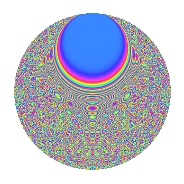# Properties

 Label 2016.2.flLevel 2016 Weight 2 Character orbit fl Rep. character $$\chi_{2016}(107,\cdot)$$ Character field $$\Q(\zeta_{24})$$ Dimension 1024 Sturm bound 768

# Related objects

## Defining parameters

 Level: $$N$$ $$=$$ $$2016 = 2^{5} \cdot 3^{2} \cdot 7$$ Weight: $$k$$ $$=$$ $$2$$ Character orbit: $$[\chi]$$ $$=$$ 2016.fl (of order $$24$$ and degree $$8$$) Character conductor: $$\operatorname{cond}(\chi)$$ $$=$$ $$672$$ Character field: $$\Q(\zeta_{24})$$ Sturm bound: $$768$$

## Dimensions

The following table gives the dimensions of various subspaces of $$M_{2}(2016, [\chi])$$.

Total New Old
Modular forms 3136 1024 2112
Cusp forms 3008 1024 1984
Eisenstein series 128 0 128

## Trace form

 $$1024q + O(q^{10})$$ $$1024q - 16q^{16} + 128q^{40} - 48q^{52} - 64q^{58} + 192q^{64} + 32q^{67} + 80q^{82} + 96q^{91} + 48q^{94} + O(q^{100})$$

## Decomposition of $$S_{2}^{\mathrm{new}}(2016, [\chi])$$ into newform subspaces

The newforms in this space have not yet been added to the LMFDB.

## Decomposition of $$S_{2}^{\mathrm{old}}(2016, [\chi])$$ into lower level spaces

$$S_{2}^{\mathrm{old}}(2016, [\chi]) \cong$$ $$S_{2}^{\mathrm{new}}(672, [\chi])$$$$^{\oplus 2}$$

## Hecke characteristic polynomials

There are no characteristic polynomials of Hecke operators in the database